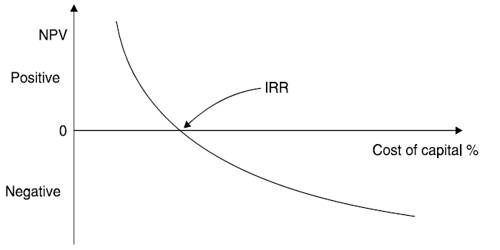# Difference between NPV and IRR

Net Present Value (NPV) is the difference between the present value of cash inflows and the present value of cash outflows. NPV is used in capital budgeting to analyze the profitability of an investment or project.

The internal rate of return, or IRR, is one of the most popular methods of evaluating potential projects. The discount rate often used in capital budgeting that makes the net present value of all cash flows from a particular project equal to zero.

Different between NPV and IRR: The difference between NPV and IRR are given below:

NPV

1. Net present value (NPV) discounts the stream of expected cash flows associated with a proposed project to their current value, which presents a cash surplus or loss for the project.
2. NPV refers to netting the present value of the initial investment.
3. Here cash inflows are conventional.
4. The cost of capital is considered discount rate at the NPV method.
5. In this method, if the net present value of a project is mite that is accepted.
6. It is easy than IRR.
7. The NPV method presents an outcome that forms the foundation for an investment decision since it presents a dollar return.

IRR

1. The internal rate of return (IRR) calculates the percentage rate of return at which those same cash flows will result in a net present value of zero.
2. IRR is defined as that discount rate that equates the PV of projects expected cash inflows with its initial cost or the present value of the outflow.
3. Here cash inflows are unconventional.
4. The discount rate is calculated by trial and error method.
5. In this method, if the IRR is greater than discount rate that is accepted
6. It is difficult than NPV.
7. The IRR method does not help in making this decision since its percentage return does not tell the investor how much money will be made.